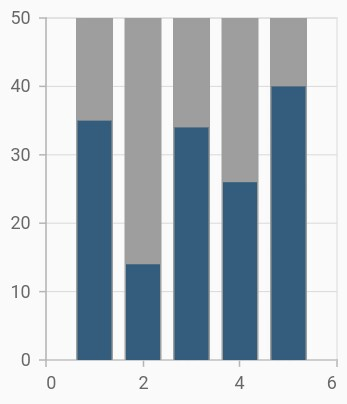# Column Chart in Flutter Cartesian Charts (SfCartesianChart)

11 Apr 202220 minutes to read

To render a column chart, create an instance of `ColumnSeries`, and add it to the `series` collection property of `SfCartesianChart`. The following properties can be used to customize the appearance:

``````@override
Widget build(BuildContext context) {
final List<ChartData> chartData = [
ChartData(1, 35),
ChartData(2, 23),
ChartData(3, 34),
ChartData(4, 25),
ChartData(5, 40)
];
return Scaffold(
body: Center(
child: Container(
child: SfCartesianChart(
series: <ChartSeries<ChartData, int>>[
// Renders column chart
ColumnSeries<ChartData, int>(
dataSource: chartData,
xValueMapper: (ChartData data, _) => data.x,
yValueMapper: (ChartData data, _) => data.y
)
]
)
)
)
);
}
class ChartData {
ChartData(this.x, this.y, this.y1);
final int x;
final double y;
}``````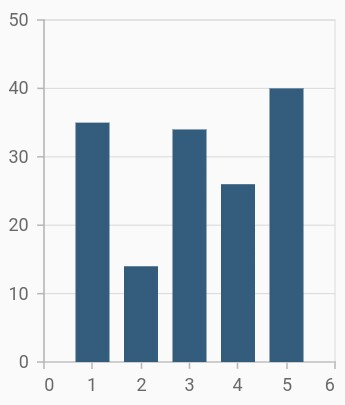## Side-by-side series placement

By default, all the column series that have the same x and y-axes are placed side by side in a chart. If you want to place a series one over the other (overlapped), set the `enableSideBySideSeriesPlacement` property of `SfCartesianChart` to false and configure the `width` property to differentiate the series. The following code snippet and screenshot illustrate the overlapped placement of column series.

``````@override
Widget build(BuildContext context) {
final List<ChartData> chartData = [
ChartData(2010, 35, 23),
ChartData(2011, 38, 49),
ChartData(2012, 34, 12),
ChartData(2013, 52, 33),
ChartData(2014, 40, 30)
];

return Scaffold(
body: Center(
child: Container(
child: SfCartesianChart(
// Columns will be rendered back to back
enableSideBySideSeriesPlacement: false,
series: <ChartSeries<ChartData, int>>[
ColumnSeries<ChartData, int>(
dataSource: chartData,
xValueMapper: (ChartData data, _) => data.x,
yValueMapper: (ChartData data, _) => data.y
),
ColumnSeries<ChartData, DateTime>(
opacity: 0.9,
width: 0.4,
dataSource: chartData,
xValueMapper: (ChartData data, _) => data.x,
yValueMapper: (ChartData data, _) => data.y1
)
]
)
)
)
);
}
class ChartData {
ChartData(this.x, this.y, this.y1);
final int x;
final double y;
final double y1;
}``````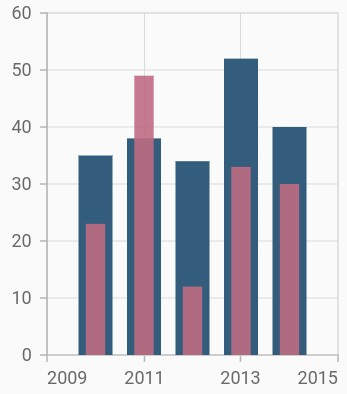## Column width and spacing

The `spacing` property is used to change the spacing between two segments. The default value of spacing is 0, and the value ranges from 0 to 1. Here, 1 and 0 correspond to 100% and 0% of the available space, respectively.

The `width` property is used to change the width of the rectangle. The default value of the width is 0.7, and the value ranges from 0 to 1. Here, 1 and 0 correspond to 100% and 0% of the available width, respectively.

``````@override
Widget build(BuildContext context) {
final List<ChartData> chartData = [
ChartData(1, 35),
ChartData(2, 23),
ChartData(3, 34),
ChartData(4, 25),
ChartData(5, 40)
];
return Scaffold(
body: Center(
child: Container(
child: SfCartesianChart(
series: <ChartSeries<ChartData, int>>[
ColumnSeries<ChartData, int>(
dataSource: chartData,
xValueMapper: (ChartData data, _) => data.x,
yValueMapper: (ChartData data, _) => data.y,
// Width of the columns
width: 0.8,
// Spacing between the columns
spacing: 0.2
)
]
)
)
)
);
}``````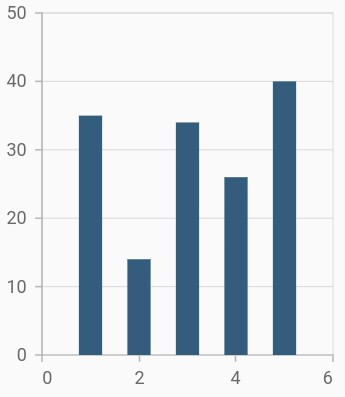## Rounded corners

The `borderRadius` property is used to add the rounded corners to the rectangle.

``````@override
Widget build(BuildContext context) {
final List<ChartData> chartData = [
ChartData(1, 35),
ChartData(2, 23),
ChartData(3, 34),
ChartData(4, 25),
ChartData(5, 40)
];
return Scaffold(
body: Center(
child: Container(
child: SfCartesianChart(
series: <ChartSeries<ChartData, int>>[
ColumnSeries<ChartData, int>(
dataSource: chartData,
xValueMapper: (ChartData data, _) => data.x,
yValueMapper: (ChartData data, _) => data.y,
)
]
)
)
)
);
}``````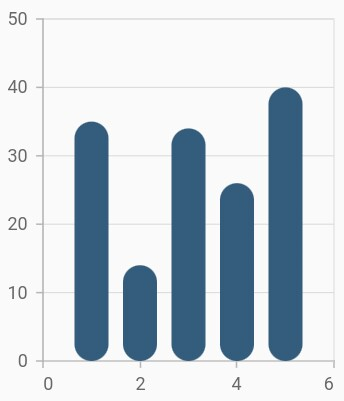## Track customization

Renders column with track. Track is a rectangular bar rendered from the start to the end of the axis. Column series will be rendered above the track. The `isTrackVisible` property is used to enable the track. The following properties can be used to customize the appearance:

``````@override
Widget build(BuildContext context) {
final List<ChartData> chartData = [
ChartData(1, 35),
ChartData(2, 23),
ChartData(3, 34),
ChartData(4, 25),
ChartData(5, 40)
];
return Scaffold(
body: Center(
child: Container(
child: SfCartesianChart(
series: <ChartSeries<ChartData, int>>[
ColumnSeries<ChartData, double>(
dataSource: chartData,
// Renders the track
isTrackVisible: true,
xValueMapper: (ChartData data, _) => data.x,
yValueMapper: (ChartData data, _) => data.y
)
]
)
)
)
);
}``````